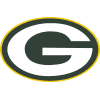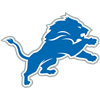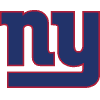DraftKings 2019 NFC Championship Odds
+185
1.85 to 1Saints
29.1% implied probability

+250
2.5 to 149ers
23.7% implied probability

+400
4 to 1Seahawks
16.6% implied probability

+650
6.5 to 1Packers
11.1% implied probability

+1200
12 to 1Vikings
6.4% implied probability

+1800
18 to 1Cowboys
4.4% implied probability

+2200
22 to 1Eagles
3.6% implied probability

+5000
50 to 1Bears
1.6% implied probability

+5000
50 to 1Rams
1.6% implied probability

+25000
250 to 1Falcons
0.3% implied probability

+25000
250 to 1Panthers
0.3% implied probability

+25000
250 to 1Lions
0.3% implied probability

+25000
250 to 1Buccaneers
0.3% implied probability

+50000
500 to 1Cardinals
0.2% implied probability

+50000
500 to 1Giants
0.2% implied probability

+50000
500 to 1Redskins
0.2% implied probability